# How to Calculation nth Root – Excel & Google Sheets

This tutorial will teach you how to calculate the nth root of a number in Excel and Google Sheets.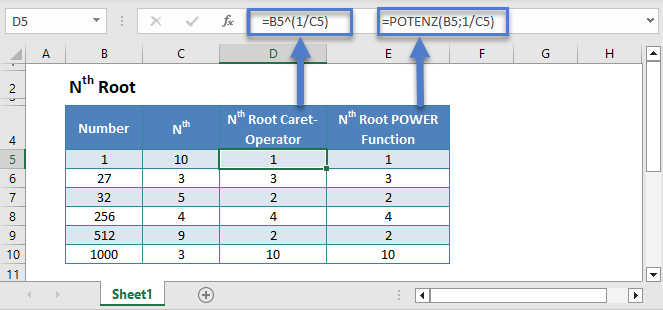## Calculating nth Root using Caret Operator (^)

We can find the nth root of a number by raising that number to the exponential ‘1/N’. To calculate the nth root of a number you can either use the Caret Operator (^) or the POWER function.

The Caret Operator can be used in the following manner:

``=B3^(1/C3)``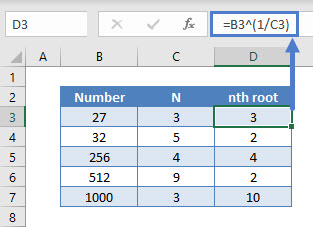The Caret Operator raises a number to power, by using a number between 0 and 1, we can take the root of the number. As shown in the first example above, by raising a number to 1/3, we can take the cube root of a number.

Note: Make sure ‘1/N’ is in parentheses to control the order of operations

## Calculating nth Root using POWER Function

Alternatively, the nth root of a number can be calculated using the POWER function. Its syntax is:

``=POWER(B3,1/C3)``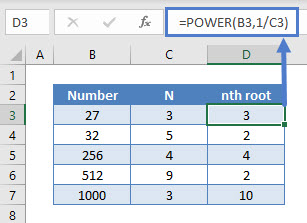## Calculating nth Root of a Negative Number using ABS Function

In case the number is negative and N is an even number, the ABS function must be used to avoid the #NUM! error. The ABS function returns the absolute value of a negative number. Its syntax is:

``=ABS(B3)^(1/C3)``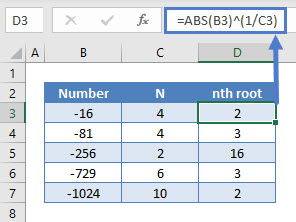In this example, the ABS function has been paired up with the Caret Operator (^) to find the nth root. It can also be nested in the POWER function in a similar manner.

## Nth Root in Google Sheets

These formulas work exactly the same in Google Sheets as in Excel.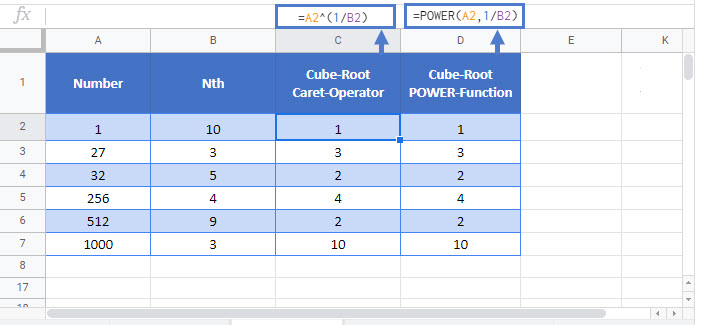### Excel Practice Worksheet

Practice Excel functions and formulas with our 100% free practice worksheets!